# Heptagon

Regular heptagon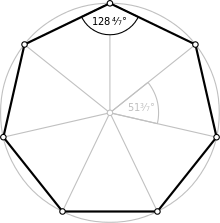A regular heptagon
Type Regular polygon
Edges and vertices 7
Schläfli symbol {7}
Coxeter diagramSymmetry group Dihedral (D7), order 2×7
Internal angle (degrees) ≈128.571°
Dual polygon Self
Properties Convex, cyclic, equilateral, isogonal, isotoxal

In geometry, a heptagon is a seven-sided polygon or 7-gon.

The heptagon is also occasionally referred to as the septagon, using "sept-" (an elision of septua-, a Latin-derived numerical prefix, rather than hepta-, a Greek-derived numerical prefix) together with the Greek suffix "-agon" meaning angle.

## Regular heptagon

A regular heptagon, in which all sides and all angles are equal, has internal angles of 5π/7 radians (128.5714286 degrees). Its Schläfli symbol is {7}.

### Area

The area (A) of a regular heptagon of side length a is given by:This can be seen by subdividing the unit-sided heptagon into seven triangular "pie slices" with vertices at the center and at the heptagon's vertices, and then halving each triangle using the apothem as the common side. The apothem is half the cotangent ofand the area of each of the 14 small triangles is one-fourth of the apothem.

The exact algebraic expression, starting from the cubic polynomial x3 + x2 − 2x − 1 (one of whose roots is) is given in complex numbers by:in which the imaginary parts offset each other leaving a real expression. This expression cannot be algebraically rewritten without complex components, since the indicated cubic function is casus irreducibilis.

The area of a regular heptagon inscribed in a circle of radius R iswhile the area of the circle itself isthus the regular heptagon fills approximately 0.8710 of its circumscribed circle.

### Construction

As 7 is a Pierpont prime but not a Fermat prime, the regular heptagon is not constructible with compass and straightedge but is constructible with a marked ruler and compass. This type of construction is called a neusis construction. It is also constructible with compass, straightedge and angle trisector. The impossibility of straightedge and compass construction follows from the observation thatis a zero of the irreducible cubic x3 + x2 − 2x − 1. Consequently this polynomial is the minimal polynomial of 2cos(7), whereas the degree of the minimal polynomial for a constructible number must be a power of 2.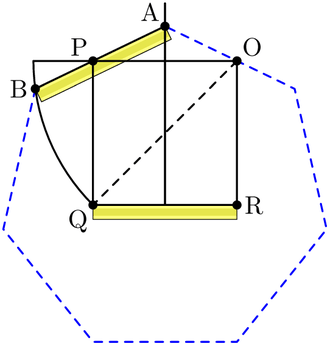A neusis construction of the interior angle in a regular heptagon.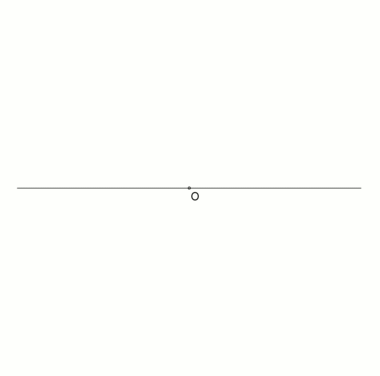An animation from a neusis construction with radius of circumcircle, according to Andrew M. Gleason based on the angle trisection by means of the Tomahawk.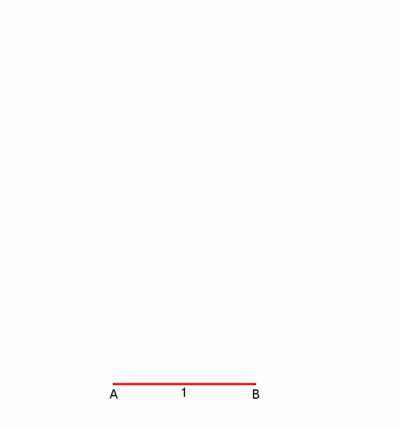Heptagon with given side length:
An animation from a neusis construction with marked ruler, according to David Johnson Leisk (Crockett Johnson)

### Approximation

An approximation for practical use with an error of about 0.2% is shown in the drawing. It is attributed to Albrecht Dürer. Let A lie on the circumference of the circumcircle. Draw arc BOC. Thengives an approximation for the edge of the heptagon.

Example to illustrate the error:
At a circumscribed circle radius r = 1 m, the absolute error of the 1st side would be approximately -1.7 mm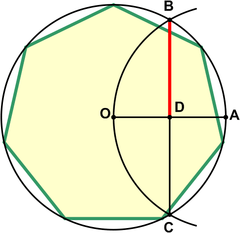### Symmetry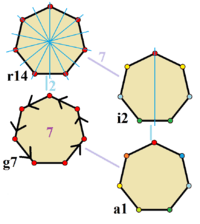Symmetries of a regular heptagon. Vertices are colored by their symmetry positions. Blue mirror lines are drawn through vertices and edges. Gyration orders are given in the center.

The regular heptagon has Dih7 symmetry, order 14. Since 7 is a prime number there is one subgroup with dihedral symmetry: Dih1, and 2 cyclic group symmetries: Z7, and Z1.

These 4 symmetries can be seen in 4 distinct symmetries on the heptagon. John Conway labels these by a letter and group order. Full symmetry of the regular form is r14 and no symmetry is labeled a1. The dihedral symmetries are divided depending on whether they pass through vertices (d for diagonal) or edges (p for perpendiculars), and i when reflection lines path through both edges and vertices. Cyclic symmetries in the middle column are labeled as g for their central gyration orders.

Each subgroup symmetry allows one or more degrees of freedom for irregular forms. Only the g7 subgroup has no degrees of freedom but can seen as directed edges.

### Diagonals and heptagonal triangle

The regular heptagon's side a, shorter diagonal b, and longer diagonal c, with a<b<c, satisfy:Lemma 1(the optic equation)

and henceand:Coro. 2Thus –b/c, c/a, and a/b all satisfy the cubic equationHowever, no algebraic expressions with purely real terms exist for the solutions of this equation, because it is an example of casus irreducibilis.

We also haveandA heptagonal triangle has vertices coinciding with the first, second, and fourth vertices of a regular heptagon (from an arbtrary starting vertex) and anglesandThus its sides coincide with one side and two particular diagonals of the regular heptagon.

## Star heptagons

Two kinds of star heptagons can be constructed from regular heptagons, labeled by Schläfli symbols {7/2}, and {7/3}, with the divisor being the interval of connection.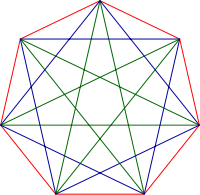Blue, {7/2} and green {7/3} star heptagons inside a red heptagon.

## Uses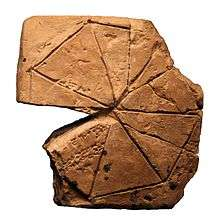Geometry problem of the surface of a heptagon divided into triangles, on a clay tablet belonging to a school for scribs; Susa, first half of the 2nd millennium BCE

The United Kingdom currently (2011) has two heptagonal coins, the 50p and 20p pieces, and the Barbados Dollar is also heptagonal. The 20-eurocent coin has cavities placed similarly. Strictly, the shape of the coins is a Reuleaux heptagon, a curvilinear heptagon to make them curves of constant width: the sides are curved outwards so that the coin will roll smoothly in vending machines. Botswana pula coins in the denominations of 2 Pula, 1 Pula, 50 Thebe and 5 Thebe are also shaped as equilateral-curve heptagons. Coins in the shape of Reuleaux heptagons are also in circulation in Mauritius, U.A.E., Tanzania, Samoa, Papua New Guinea, São Tomé and Príncipe, Haiti, Jamaica, Liberia, Ghana, the Gambia, Jordan, Jersey, Guernsey, Isle of Man, Gibraltar, Guyana, Solomon Islands, Falkland Islands and Saint Helena. The 1000 Kwacha coin of Zambia is a true heptagon.

The Brazilian 25-cent coin has a heptagon inscribed in the coin's disk. Some old versions of the coat of arms of Georgia, including in Soviet days, used a {7/2} heptagram as an element.

In architecture, heptagonal floor plans are very rare. A remarkable example is the Mausoleum of Prince Ernst in Stadthagen, Germany.

Many police badges in the US have a 7:2 heptagram outline.

Apart from the heptagonal prism and heptagonal antiprism, no convex polyhedron made entirely out of regular polygons contains a heptagon as a face.

Regular heptagons can tile the hyperbolic plane, as shown in this Poincaré disk model projection: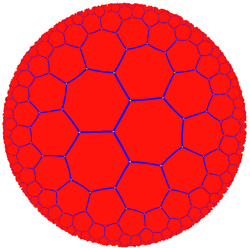heptagonal tiling

## Graphs

The K7 complete graph is often drawn as a regular heptagon with all 21 edges connected. This graph also represents an orthographic projection of the 7 vertices and 21 edges of the 6-simplex. The regular skew polygon around the perimeter is called the petrie polygon.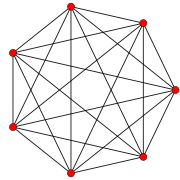6-simplex (6D)

## References

1. Gleason, Andrew Mattei (March 1988). "Angle trisection, the heptagon, and the triskaidecagon p. 186 (Fig.1) –187" (PDF). The American Mathematical Monthly. 95 (3): 185–194. doi:10.2307/2323624. Archived from the original (PDF) on 2015-12-19.
2. Weisstein, Eric W. "Heptagon." From MathWorld, A Wolfram Web Resource.
3. G.H. Hughes, "The Polygons of Albrecht Dürer-1525, The Regular Heptagon", Fig. 11 the side of the Heptagon (7) Fig. 15, image on the left side, retrieved on 4th December 2015
4. John H. Conway, Heidi Burgiel, Chaim Goodman-Strauss, (2008) The Symmetries of Things, ISBN 978-1-56881-220-5 (Chapter 20, Generalized Schaefli symbols, Types of symmetry of a polygon pp. 275-278)
5. Abdilkadir Altintas, "Some Collinearities in the Heptagonal Triangle", Forum Geometricorum 16, 2016, 249–256.http://forumgeom.fau.edu/FG2016volume16/FG201630.pdf
6. Leon Bankoff and Jack Garfunkel, "The heptagonal triangle", Mathematics Magazine 46 (1), January 1973, 7–19.Look up Heptagon in Wiktionary, the free dictionary.